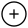## Ultimate Guide to solve almost any Coding problem with any level of difficultly

16 March, 2022

If you are participating in any Coding Competitions or preparing for Coding interviews, or just practicing Coding problems on any coding platform and want to save some time in thinking of an efficient approach after seeing the coding question, then you are at the right place.

Below is a simple and easy-to-understand yet useful guide on what approach to take when you first see a certain type of problem. Of course, this is not relevant every time, but most of the time, this really works.

Consider the below instructions as a conclusion after solving more than a thousand of a variety of coding problems online.

So, here it goes:

If the input array is sorted then try using:

• Binary search
• Two pointers

If you must solve in-place then try using:

• Swap corresponding values
• Store one or more different values in the same pointer
• Check if it is written in questions like "Inplace excluding input and output variables". If it is so, then try to use the resultant variable. For example, using a resultant array like a map

If asked for all permutations/subsets then try using:

• Backtracking

If given a tree or graph then try using:

• DFS
• BFS

If given a linked list then try using:

• Two-way pointers
• Faster and Slower pointer

If recursion is banned then try:

• Use Array as Stack

If asked for maximum/minimum of subarray/subset then try using:

• Dynamic programming

I asked for top/bottom/least K items then try using:

• Heap

If asked for common strings then try using:

• Map
• Trie

If optimization is needed in the case of string/arrays then try:

• Map/Set as it has O(1) time & O(n) space
• Try to sort input for O(N log N) time and O(1) space

Hope this helps. Also please don't forget to comment on any other tips you want to add to this guide. Thank you.

Programming Competitive Programming competitivecoding coding Programming Codechef Leetcode Hackerrank Codeforces